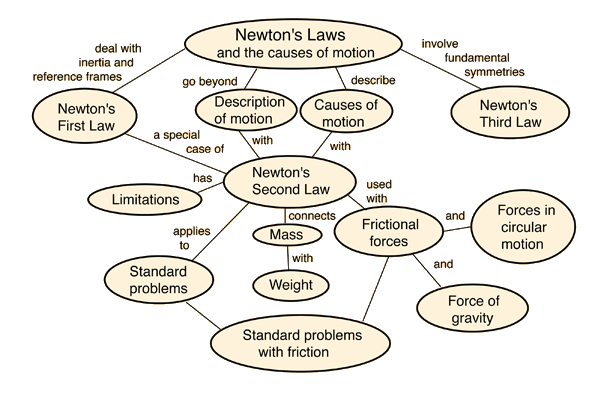Index

 HyperPhysics***** Mechanics R Nave
Go Back

# Newton's First Law

Newton's First Law states that an object will remain at rest or in uniform motion in a straight line unless acted upon by an external force. It may be seen as a statement about inertia, that objects will remain in their state of motion unless a force acts to change the motion. Any change in motion involves an acceleration, and then Newton's Second Law applies. The First Law could be viewed as just a special case of the Second Law for which the net external force is zero, but that carries some presumptions about the frame of reference in which the motion is being viewed. The statements of both the Second Law and the First Law here are presuming that the measurements are being made in a reference frame which is not itself accelerating. Such a frame is often referred to as an "inertial frame". The statement of these laws must be generalized if you are dealing with a rotating reference frame or any frame which is accelerating.

Newton's First Law contains implications about the fundamental symmetry of the universe in that a state of motion in a straight line must be just as "natural" as being at rest. If an object is at rest in one frame of reference, it will appear to be moving in a straight line to an observer in a reference frame which is moving by the object. There is no way to say which reference frame is "special", so all constant velocity reference frames must be equivalent.

Index

Mass on string example.

Newton's laws concepts.

 HyperPhysics***** Mechanics R Nave
Go Back

# Centripetal Force Example

The string must provide the necessary centripetal force to move the ball in a circle. If the string breaks, the ball will move off in a straight line. The straight line motion in the absence of the constraining force is an example of Newton's first law. The example here presumes that no other net forces are acting, such as horizontal motion on a frictionless surface. The vertical circle is more involved.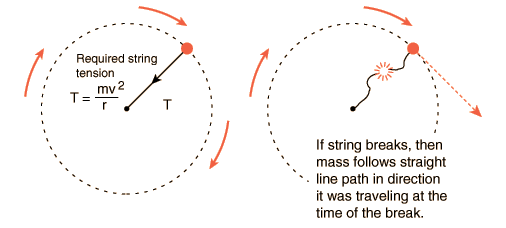Centripetal force calculation
Index

 HyperPhysics***** Mechanics R Nave
Go Back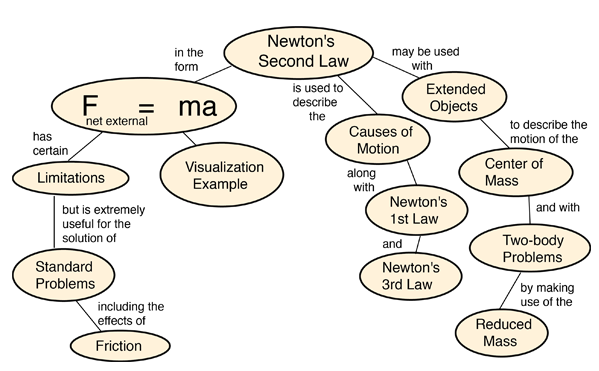Index

Newton's laws concepts.

 HyperPhysics***** Mechanics R Nave
Go Back

# Newton's Second Law

Newton's Second Law as stated below applies to a wide range of physical phenomena, but it is not a fundamental principle like the Conservation Laws. It is applicable only if the force is the net external force. It does not apply directly to situations where the mass is changing, either from loss or gain of material, or because the object is traveling close to the speed of light where relativistic effects must be included. It does not apply directly on the very small scale of the atom where quantum mechanics must be used.

Data can be entered into any of the boxes below. Specifying any two of the quantities determines the third. After you have entered values for two, click on the text representing the third to calculate its value.Newtons = kg * m/s2
pounds = slugs * ft/s2
 Limitations of Newton's Second Law
Index

Second Law Concepts

Visualization example.

 HyperPhysics***** Mechanics R Nave
Go Back

# Newton's Second Law Illustration

Newton's 2nd Law enables us to compare the results of the same force exerted on objects of different mass.Index

 HyperPhysics***** Mechanics R Nave
Go Back

# Newton's Third Law

Newton's third law: All forces in the universe occur in equal but oppositely directed pairs. There are no isolated forces; for every external force that acts on an object there is a force of equal magnitude but opposite direction which acts back on the object which exerted that external force. In the case of internal forces, a force on one part of a system will be countered by a reaction force on another part of the system so that an isolated system cannot by any means exert a net force on the system as a whole. A system cannot "bootstrap" itself into motion with purely internal forces - to achieve a net force and an acceleration, it must interact with an object external to itself.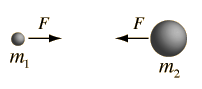Without specifying the nature or origin of the forces on the two masses, Newton's 3rd law states that if they arise from the two masses themselves, they must be equal in magnitude but opposite in direction so that no net force arises from purely internal forces.

Newton's third law is one of the fundamental symmetry principles of the universe. Since we have no examples of it being violated in nature, it is a useful tool for analyzing situations which are somewhat counter-intuitive. For example, when a small truck collides head-on with a large truck, your intuition might tell you that the force on the small truck is larger. Not so!

 Small truck,large truck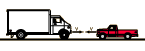Example of Newton's Third Law
Index

Example

Newton's laws concepts.

 HyperPhysics***** Mechanics R Nave
Go Back

# Newton's Third Law Example

Newton's third law can be illustrated by identifying the pairs of forces which are involved in supporting the blocks on the spring scale.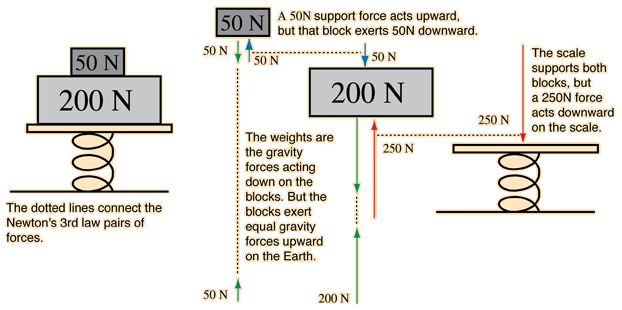Presuming that the blocks are supported and at equilibrium, then the net force on the system is zero. All the forces occur in Newton's third law pairs.

Index

 HyperPhysics***** Mechanics R Nave
Go Back